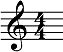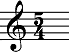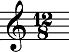## Understanding Meter and its Application Part I

### SO, WHAT IS METER?

Meters, or time signatures, are ways of organizing music. They help musicians in numerous ways, but are commonly overlooked. Meter organizes music by phrase, rhythm, or by chord changes. Specifically, they organize the rhythm of the music.

There are two parts to any time signature: a top number and a bottom number. The top number tells you "how many" while the bottom number tells you "of what." The top number indicates how many notes there will be per measure and the bottom number indicates what kind of note the top number is referring to.

This example would have four quarter note beats per measure:This example would have five quarter note beats per measure:This example would have twelve eighth note beats per measure:The groupings in a time signature refer to how the rhythms can be divided. The rhythms are divided into groups of twos or threes. Look at the notation below to visualize this:Notice how the first measure is completely grouped as 2+2+2+2 while the second measure is grouped as 3+3+2. There are two main reasons that groupings are important. One, it is easier forthe performer to visualize how the passage should be played. Two, it gives an accurate descriptionof how the passage should be performed. For the most part, the first note of each grouping is given the accent. This means it will be dynamically accented more than the following notes of the same grouping.

### SIMPLE METER

A simple meter is a time signature where each beat isdivided into two parts. Common examples of simple meter include 2/4, 3/4 and 4/4. Pay attention to the 8th notes in the following examples.

This example is in 3/4 meter:### COMPOUND METER

A compound meter is a time signature where each beat is divided into three parts. Common examples of compound meter include 6/8, 9/8 and 12/8. The common beat or pulse in compound meter is a dotted quarter note. Each dotted quarter note is divided into three eighth notes.

This example is in 9/8 meter:### ODD METER

An odd meter is a time signature that includes both simple and compound meter. This happens due to the odd number notes indicated by the top number of the meter. Let's use 7/8 as an example. 7/8 can be divided a few different ways

This example is in 7/8 meter: